# Python Program to Print Star, Pyramid, Number Patterns

In this article, you will learn and get code in Python, to print pattern of stars (*), numbers, alphabets. There are many pattern programs available in this article:

• Half Pyramid of Stars (*)
• Inverted Half Pyramid of *
• Full Pyramid of *
• Inverted Full Pyramid of *
• Pyramid of Given Size by user at run-time
• Print Pattern of * using Function
• Print Pattern based on User's Choice
• Pattern of *
• Pattern of Numbers
• Pattern of Alphabets

To print pyramid pattern of stars, numbers, or alphabets in python, you have to use two or more for loops. In most of the program that contains two for loops. The first loop corresponds to rows, whereas the second loop corresponds to columns.

## Print Pyramid Pattern of Stars (*)

Let's start with pyramid pattern of stars (*). In this section, there are more than 6 programs. All programs prints pyramid pattern of stars, but in different-different ways. Let's have a look at these programs one by one.

### Half Pyramid of Stars (*)

This program prints half-pyramid of stars (*) of 5 lines. That is, one star in first row, two stars in second row, three stars in third row, and so on, upto five stars in fifth row. All stars starting from first column, or from left side:

```print("Half Pyramid Pattern of Stars (*):")
for i in range(5):
for j in range(i+1):
print("* ", end="")
print()```

Here is the sample run of the above python program to illustrates how to print pattern using stars:

In first row you will see only 1 star, in second row you will see 2 stars and so on upto 5th row. Printing of stars starts from very first column in every row as shown in the snapshot given below: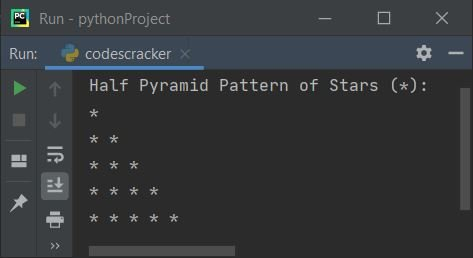Note - The range() function returns a sequence of values. By default, the value starts with 0 and increments by 1. It stops before a number specified as argument of the function.

For example, the following block of code:

```for i in range(5):
print(i)```

prints:

```0
1
2
3
4```

Therefore in above program, the following code:

`for i in range(5):`

is used to execute the following block of code:

```for j in range(i+1):
print("* ", end="")
print()```

five number of times with value of i from 0 to 4. The following statement:

`print("* ", end="")`

can also be written as:

`print(end="* ")`

Both statements prints a * and a space on output. Here we've used end, because to skip newline for next output thing. That is, the statement print() prints the thing inside its braces and a newline automatically gets printed after printing the thing given in its braces. For example, the following statement:

`print()`

is used to print newline, that is, after executing this statement, next thing starts from newline. So we've used end to end with the thing that assigned to it. The second for loop in above program, that is:

`for j in range(i+1):`

is created to run the following statement:

`print("* ", end="")`

i+1th number of times with value from 0 to i.

### Inverted Half Pyramid of Stars (*)

This python program prints pattern of stars (*) that looks like an inverted half pyramid:

```print("Inverted Half Pyramid of Stars (*):")
for i in range(5):
for j in range(i, 5):
print("* ", end="")
print()```

Here is the output produced by this python program: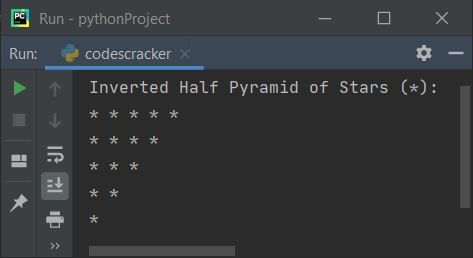### Full Pyramid of Stars (*)

This program prints pattern of stars that looks like a pyramid:

```print("Full Pyramid Pattern of Stars (*): ")
for i in range(5):
for s in range(-6, -i):
print(" ", end="")
for j in range(i+1):
print("* ", end="")
print()```

Here is its sample output, the pyramid pattern of stars: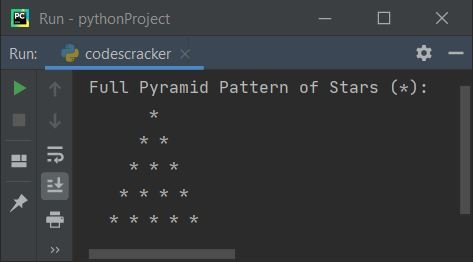### Inverted Full Pyramid of Stars (*)

This is the inverse of previous program. That is, this program prints an inverted pyramid of stars:

```print("Inverted Full Pyramid of Stars (*): ")
for i in range(5):
for s in range(i):
print(" ", end="")
for j in range(i, 5):
print("* ", end="")
print()```

The snapshot given below shows the sample output produced by this python program:### Print Star Pyramid of Given Size

Now this program allows user to define the size of pyramid. For example, if user enters 10 as input, then the program prints star pyramid of 10 rows or lines:

```print("Enter Number of Rows: ")
row = int(input())
print("Star Pyramid of " + str(row) + " Rows or Lines: ")
for i in range(row):
for s in range(row, i, -1):
print(end=" ")
for j in range(i+1):
print(end="* ")
print()```

Here is the initial output produced by this python program:Now supply the input say 8 and print `ENTER` key to see the following output, the star pyramid of 8 lines: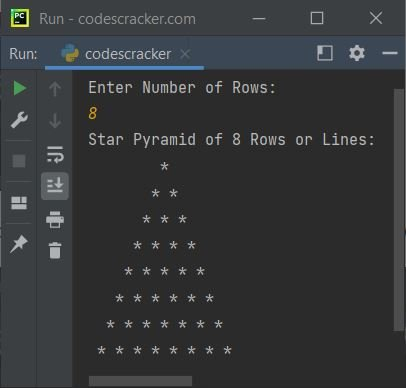Note - The str(row) is used to convert the value of row (an integer value) to string. Because addition of string and integer using + gives you an error.

### Four Pyramid Patterns of * using Function

Let's create a program using user-defined functions to print all the four pyramid shapes of *:

```def halfPyramid():
for i in range(5):
for j in range(i+1):
print(end="* ")
print()
def invertedHalfPyramid():
for i in range(5):
for j in range(i, 5):
print(end="* ")
print()
def fullPyramid():
for i in range(5):
for s in range(5, i+1, -1):
print(end=" ")
for j in range(i + 1):
print(end="* ")
print()
def invertedFullPyramid():
for i in range(5):
for s in range(i):
print(end=" ")
for j in range(i, 5):
print(end="* ")
print()
halfPyramid()
print("------------------------")
invertedHalfPyramid()
print("------------------------")
fullPyramid()
print("------------------------")
invertedFullPyramid()```

In above program, we've defined all the four functions corresponds to print four pattern of pyramid. Then called all the four functions to do the task one by one. Here is its sample output: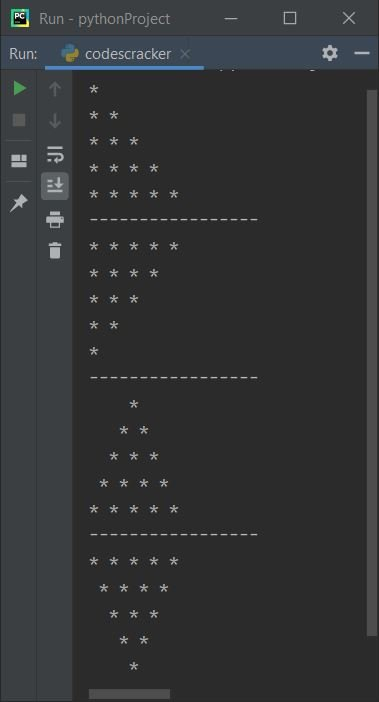### Pyramid of Stars based on User's Choice

Now this is a menu-driven program that allows user to enter the choice to print desired pattern. The menu continuously gets displayed, until user wants to exit. Let's have a look at the program and its output to understand it in better way:

```def halfPyramid():
for i in range(5):
for j in range(i+1):
print(end="* ")
print()
def invertedHalfPyramid():
for i in range(5):
for j in range(i, 5):
print(end="* ")
print()
def fullPyramid():
for i in range(5):
for s in range(5, i+1, -1):
print(end=" ")
for j in range(i + 1):
print(end="* ")
print()
def invertedFullPyramid():
for i in range(5):
for s in range(i):
print(end=" ")
for j in range(i, 5):
print(end="* ")
print()
while True:
print("1. Print Half Pyramid of Stars")
print("2. Print Inverted Half Pyramid of Stars")
print("3. Print Full Pyramid of Stars")
print("4. Print Inverted Full Pyramid of Stars")
print("5. Exit")
choice = int(input())
if choice==1:
halfPyramid()
elif choice==2:
invertedHalfPyramid()
elif choice==3:
fullPyramid()
elif choice==4:
invertedFullPyramid()
elif choice==5:
print("Exiting...")
break
else:
print("Wrong Choice!")```

Here is its initial output:Now enter your choice, whatever you want to print. That is, type 1 and press `ENTER` key to print half pyramid of stars as shown in the snapshot given below:As you can see from the above sample run, all options again gets displayed to choose and print the pattern again. Here is the sample run with all options: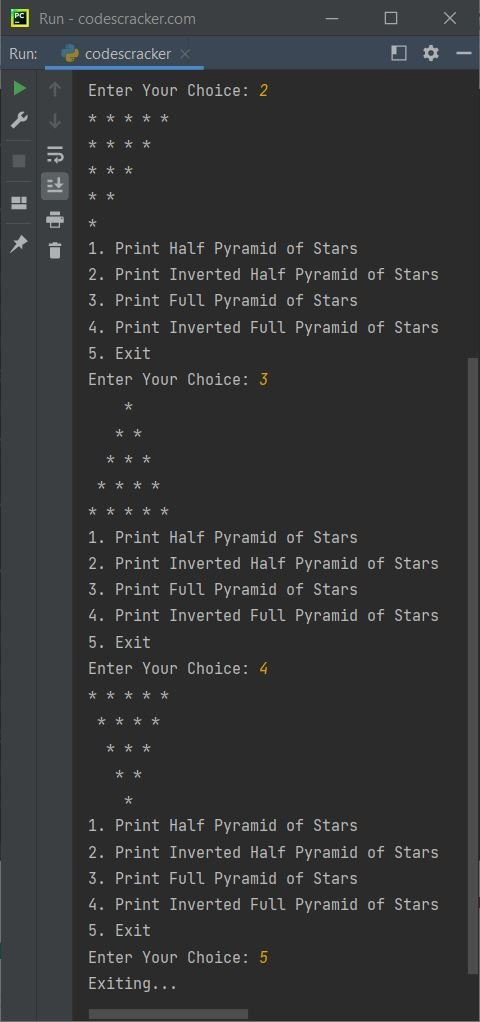In above program, when user enters a number 5 as choice, then using break keyword, the execution of while loop gets ended.

## Print Pattern of *

This program prints pattern of stars in this way:

• 1 star in first row
• 3 stars in second row
• 5 stars in third row
• and so on upto
• 9 stars in fifth row

```print("Pattern of Stars (*): ")
k = 1
for i in range(5):
for j in range(k):
print("* ", end="")
k = k + 2
print()```

Here is its sample output:This program is the reverse of previous program. That is, in every row, stars gets printed from right side:

```print("Pattern of Stars (*): ")
k = 1
space = 16
for i in range(5):
for j in range(space):
print(" ", end="")
space = space-4
for j in range(k):
print("* ", end="")
k = k + 2
print()```

The snapshot given below shows the sample output produced by this program: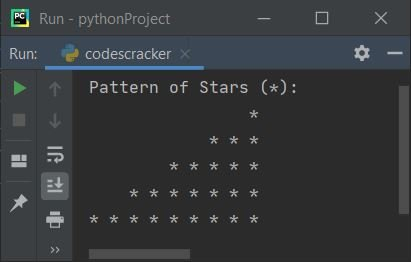Here is another program on star pattern. This program prints triangle of stars (*):

```print("Triangle Star (*) Pattern: ")
space = 8
for i in range(5):
for j in range(space):
print(" ", end="")
space = space-2
for j in range(i+1):
print("* ", end="")
print()```

Below is its sample output: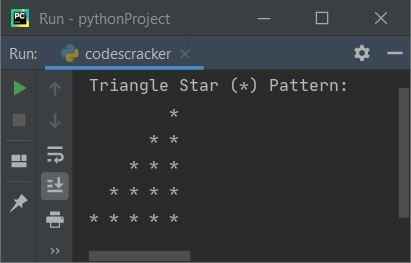## Print Pattern of Numbers

Now let's print pattern of numbers. This program prints half pyramid pattern of numbers:

```print("Pattern of Numbers: ")
num = 1
for i in range(5):
for j in range(i+1):
print(num, end=" ")
num = num+1
print()```

This is its output: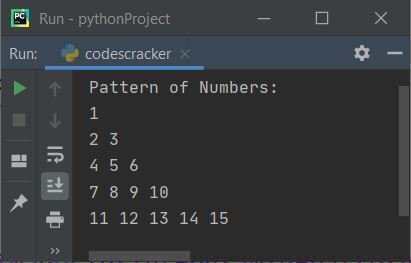### Print Pattern 1 12 123

Now let's create another program similar to previous one. The program stars with 1 from every row:

```print("Pattern of Numbers: ")
num = 1
for i in range(5):
for j in range(i+1):
print(num, end=" ")
num = num+1
num = 1
print()```

Below is its sample output: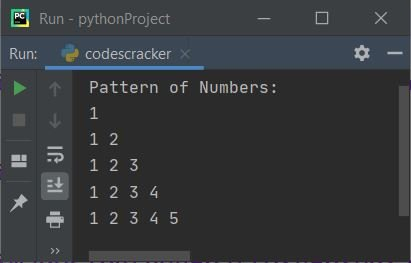### Print 12345 1234 123 12 1

Now let's create a program that prints pattern of numbers that looks like an inverted half pyramid:

```print("Pyramid Pattern of Numbers: ")
for i in range(5):
num = 1
for j in range(5, i, -1):
print(num, end=" ")
num = num + 1
print()```

This is its sample output, shows an inverted half-pyramid of numbers: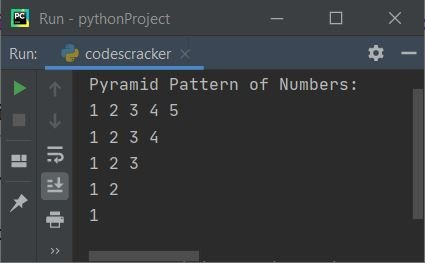This is the last program on number pattern. This program prints numbers from right side. That is, each row starts with 1 from right most side. Let's have a look at the program first, then its output to understand it in better way:

```print("Inverted Half Pyramid of Numbers: ")
decr = 8
for i in range(5):
count = 0
for k in range(decr):
print(end=" ")
decr = decr - 2
for j in range(i+1):
count = count + 1
num = count
for j in range(i+1):
print(num, end=" ")
num = num - 1
print()```

This is the output produced by above python program: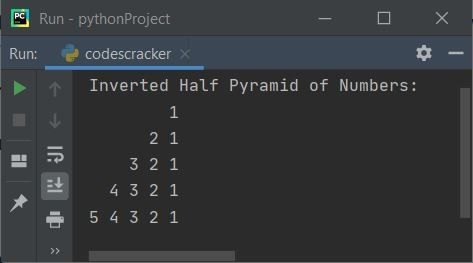## Print Pattern of Alphabets

This is the last section of this article. This section prints pattern of alphabets:

```print("Pattern of Alphabets: ")
val = 65
for i in range(5):
for j in range(i+1):
ch = chr(val)
print(ch, end=" ")
val = val+1
print()```

Here is the output of this program: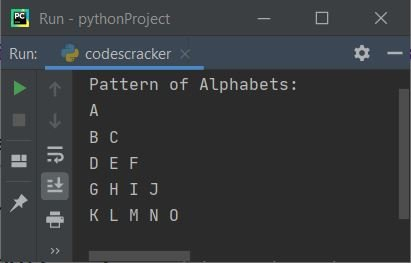Below is another program that prints half-pyramid of alphabets (A, B, C, ..):

```print("Half Pyramid Pattern of Alphabets: ")
val = 65
for i in range(5):
for j in range(i+1):
ch = chr(val)
print(ch, end=" ")
val = val+1
print()```

This program produces the output as shown in the snapshot given below: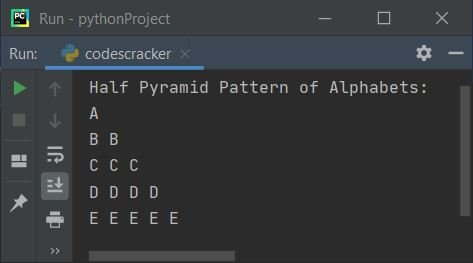This is the last program that prints an inverted half pyramid of alphabets:

```print("Inverted Half Pyramid of Alphabets: ")
decr = 8
for i in range(5):
count = 0
for k in range(decr):
print(end=" ")
decr = decr - 2
for j in range(i):
count = count + 1
num = count+65
ch = chr(num)
for j in range(i+1):
print(ch, end=" ")
num = num - 1
ch = chr(num)
print()```

Here is its sample output, an inverted half pyramid of alphabets: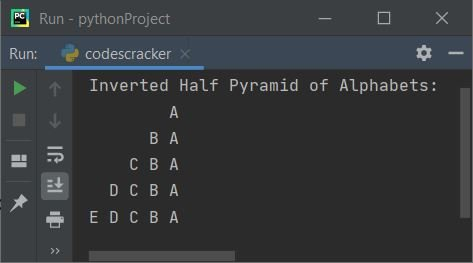#### Same Program in Other Languages

Python Online Test

« Previous Program Next Program »